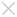## Learning

• ### White Paper

#### Happy CADing with ZW3D: Design Bronze Jue Cup with ZW3D

In this article, we will show you how to design a Bronze Jue Cup, a tripod vessel or goblet used to serve or warm wine in ancient China, with ZW3D step by step. Jue is also a royal gift for nobles and heroes from the emperor.1. Click 'Insert Sketch' from the Shape tab, select the XZ plane as the reference plane and draw a curve just like the one shown in figure 1. Exit the sketch panel to extrude this curve. While extruding, set extrude type to 'Symmetrical' with an end point of 30 (see figure 2). After that, draw a curve on the XY plane (see figure 3), then click the 'Project to Face' icon from 'Wireframe' to project it on the face shown in figure 2, the result is shown in figure 4.Figure 1                                                    Figure 2Figure 3                                                   Figure 4

1. Draw a circle with a radius of 25 on the XY plane as is shown in figure 5, then using the 'Move' feature, move this circle along the Z direction with a distance of 8, as is shown in figure 6. Next, draw 4 curves in 4 different sketches, which will make it easier to correctly mesh a face along these curves, as is shown in figure 7 to 10.Figure 5                                                    Figure 6Figure 7                                                 Figure 8Figure 9                                                      Figure 10

Finally, click the 'Curve Mesh' icon from the 'Basic Face' tool of the 'Free Form' tab, select curve 1 and curve 2 as the input of 'U curves', and the other 4 curves as the input of V curves. The result is shown in figure 11.Figure 11

1. Click the 'Extrude' icon, then select the circle under the face we just drew as the profile P, set its start as 0, end as 40, and set the draft angle as 3 with the boundary as no end caps, as is shown in figure 12. Next click 'Dome' from the 'Basic Face' tool of the 'Free Form' tab, and set a dome in the bottom of the extrude. After finishing this step, you should click 'Shell' from the 'Shape' tab to shell this model, as is shown in figure 13.Figure 12Figure 13

1. Draw the sketch shown in figure 14 in the XY plane. Next click 'Wrap patterns to faces' from the 'Morph' tool of the 'Shape' tab. The parameter of the manager is shown in figure 15. Then use the 'Pattern' feature to copy the pattern, as is shown in figure 16. Finally, click the 'Inlay' feature from the 'Edit basic' tool of the 'Shape' tab, to offset these pattern, as is shown in figure 17.Figure 14                                                Figure 15Figure 16                                                     Figure 17

1. Draw a circle as shown in figure 18, and then extrude it under the base operation, as is shown in figure 19. Click 'Fillet' to smooth the bottom line of the cylinder, as is shown in figure 20. Next, build the part shown in figure 21. After that, extrude as a short cylinder and smooth its top and button edges as is show in figure 22 and 23. Finally, mirror the parts we just drew to the other side, as is shown in figure 24.Figure 18                                                    Figure 19Figure 20                                                   Figure 21Figure 22                                                   Figure 23Figure 24

1. Draw a curve as shown in figure 25, then insert a datum plane as is shown in figure 26. Draw a trapezoid on the datum plane we just drew, as is shown in figure 27, then click 'Drive Curve Loft' to loft the leg. In order to loft the leg we should add several scale points to limit its scale along the drive curve, as is shown in figure 28.Figure 25                                                  Figure 26Figure 27                                                  Figure 28

1. Draw a sketch on the XZ plane and project it to the face shown in figure 29. Inlay this sketch into the leg using the 'Inlay' feature, as is shown in figure 30, and smooth the sketch using the 'Fillet' feature, as is shown in figure 31. Finally, embed the sketch shown in figure 32 using the same method.Figure 29                                                 Figure 30Figure 31                                               Figure 32

1. Draw a sketch like that shown in figure 33, and cut the redundant part using the 'Revolve' feature, as is shown in figure 34. Then copy this leg using the 'Pattern' feature, as is shown in figure 35.Figure 33                                             Figure 34Figure 35

1. Draw a circle just like the one shown in figure 36, then draw a cylinder using the 'Extrude' feature, as is shown in figure 37 and 38, then smooth their edge using 'Fillet', as is shown in figure 39. Finally, add texture and you will see the bronze goblet in figure 40.Figure 36                                            Figure 37Figure 38Figure 39Figure 39

ZW3D is all-in-one, affordable CAD/CAM solution that enables concept-to-finished-product designs in an integrated, collaborative environment. The proprietary Overdrive™ kernel delivers 3D part and assembly modeling, 2D production drawings, reverse engineering, motion simulation, mold design, and integrated CNC machining. It simplifies the design process from concept to completion.

Key words:

ZW3D CAD/CAM all-in-one solution, history manager, data encapsulation, history encapsulation, history regeneration, view layout, state feature, sheet metal layout

http://www.zwsoft.com

• Previous

• Next

## ·ZW3D 2016 Know-how:How to Use the Upgraded Pattern Command?2016-03-31